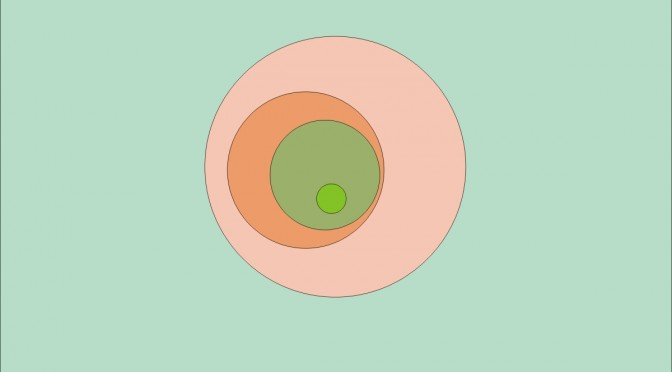# An empty intersection of nested closed convex subsets in a Banach space

We consider a decreasing sequence $$(C_n)_{n \in \mathbb{N}}$$ of non empty closed convex subsets of a Banach space $$E$$.

If the convex subsets are closed balls, their intersection is not empty. To see this let $$x_n$$ be the center and $$r_n > 0$$ the radius of the ball $$C_n$$. For $$0 \leq n < m$$ we have $$\Vert x_m-x_n\Vert \leq r_n – r_m$$ which proves that $$(x_n)_{n \in \mathbb{N}}$$ is a Cauchy sequence. As the space $$E$$ is Banach, $$(x_n)_{n \in \mathbb{N}}$$ converges to a limit $$x$$ and $$x \in \bigcap_{n=0}^{+\infty} C_n$$.

We’ll see that $$\bigcap_{n=0}^{+\infty} C_n$$ can be empty even in the case where $$d = \sup_n d(0,C_n)$$ is finite. Note: when $$\sup_n d(0,C_n)$$ is infinite, $$\bigcap_{n=0}^{+\infty} C_n$$ can be empty, i.e. if $$C_n=[n \cdot u, +\infty \cdot u )$$ where $$u \in E \setminus \{0\}$$.

Let $$E$$ be the set of real continuous functions defined on $$[0,1]$$ and $$(r_n)_{n \in \mathbb{N}}$$ an enumeration of the rational numbers of $$[0,1]$$. Let $$B=\{f \in E ; \Vert f \Vert \leq 2\}$$ and $$C=\{f \in E ; \int_0^1 f = 1\}$$. We take $$C_n=B \bigcap C \bigcap \{f \in E ; f(r_0)=f(r_1)= \dots =f(r_n)=0\}$$. For all $$n \in \mathbb{N}$$, $$C_n$$ is convex as it is the intersection of convex subsets of $$E$$. $$C_n$$ is not empty. To see this, let’s note $$q_0=0 < q_1 < q_2 < \dots < q_m =1$$ the set $$\{0,1, r_0,r_1, \dots r_n\}$$ of rational numbers and $$f_n$$ the piecewise affine function such that $$f_n(q_i)=0$$ for $$0 \leq i \leq m$$ and $$f_n(q^\prime_j)=2$$ where $$q^\prime_j$$ is arbitrarily chosen in $$(q_j,q_{j+1})$$ for $$0 \leq j \leq m-1$$. $$f_n \in C_n$$ as $$\int_0^1 f = 1$$. However, $$\bigcap_{n=0}^{+\infty} C_n$$ is empty as a function $$f$$ in the intersection has to vanish at all rational of $$[0,1]$$. Consequently, $$f$$ is the zero function, contradicting the requirement $$\int_0^1 f = 1$$.

If $$(E,\Vert \cdot \Vert)$$ is uniformly convex (in particular if $$E$$ is a Hilbert space) and $$d = \sup_n d(0,C_n)$$ is finite, then $$\bigcap_{n=0}^{+\infty} C_n$$ cannot be empty. For the proof, let $$P_n=C_n \cap B(0, d+1/n)$$. $$(P_n)$$ is a decreasing sequence of nonempty closed sets. We claim that $$\lim\limits_{n \rightarrow \infty} \delta(P_n)=0$$, where $$\delta(P_n)$$ denotes the diameter of the set $$P_n$$. Indeed, for any $$n$$, let $$x_n$$ and $$y_n$$ be arbitrary points in $$P_n$$. Since $$P_n$$ is convex, the point $$(x_n+y_n)/2$$ lies in $$P_n$$, so the values $$\Vert x_n \Vert$$, $$\Vert y_n \Vert$$, and $$\frac{1}{2} \Vert x_n + y_n \Vert$$ are between $$d + 1/n$$ and $$d(0,C_n)$$, and therefore the three sequences converge to $$d$$ as $$C_n$$ is a decreasing sequence of sets. Because the norm $$\Vert \cdot \Vert$$ is uniformly convex, we infer that $$\Vert x_n – y_n \Vert \rightarrow 0$$, and consequently $$\lim\limits_{n \rightarrow \infty} \delta(P_n)=0$$. $$(P_n)$$ is a decreasing sequence of nonempty closed sets of diameters converging to $$0$$ in a Banach space, so $$\bigcap_{n=0}^{+\infty} P_n$$ is nonempty and therefore $$\bigcap_{n=0}^{+\infty} C_n$$ is also nonempty.